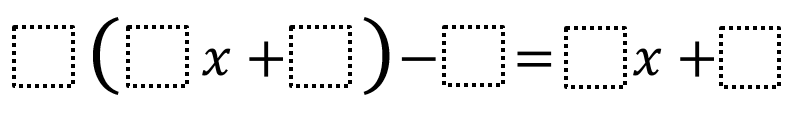Home > Grade 6 > Expressions & Equations > Equivalent Expressions 2

# Equivalent Expressions 2

Directions:  Using the whole numbers 1-9, fill in the boxes to create two equivalent expressions. You may use each whole number at most once.### Hint

How does subtracting a number affect the left side of the equation?

There are many possibilities, here are some:

3(2x + 4) – 5 = 6x + 7
3(2x + 4) – 7 = 6x + 5
3(2x + 5) – 7 = 6x + 8
3(2x + 5) – 8 = 6x + 7
2(4x + 3) – 1 = 8x + 5
2(4x + 3) – 5 = 8x + 1
2(3x + 4) – 1 = 6x + 7
2(3x + 4) – 7 = 6x + 1

Source:  Will Case

## Subtracting Multi-Decimals

Directions: Use the digits 1 to 9, at most one time each, to fill in …